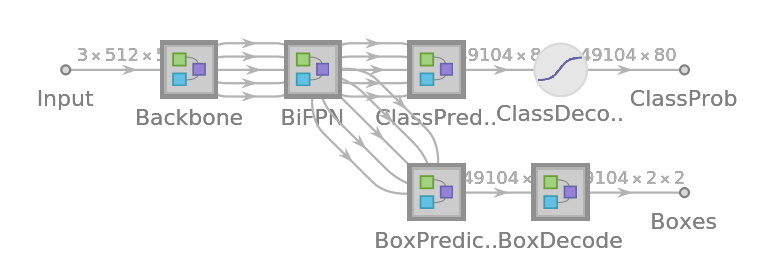# EfficientDet Trained onMS-COCO Data

Detect and localize objects in an image

Released in 2020, this family of object detection models is obtained by uniformly scaling the resolution, depth, and width of the original EfficientNet models, obtaining larger nets. In addition, a novel weighted bidirectional feature pyramid network (BiFPN) is introduced for fast and easy multiscale feature fusion. EfficientDet-D7 achieves stateof-the-art 55.1 AP on COCO test-dev, being 4x – 9x smaller and using 13x – 42x fewer FLOPs than previous detectors.

Number of models: 9

## Training Set Information

• Microsoft COCO, a dataset for image recognition, segmentation and captioning, consisting of more than three hundred thousand images overall consisting of 80 object classes.

## Performance

• The models achieve the following accuracies on the MS-COCO test set.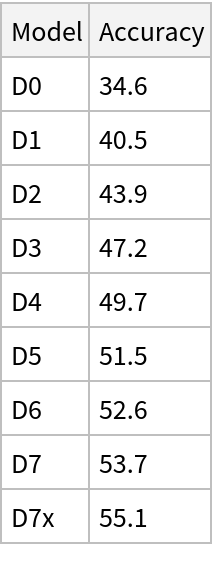## Examples

### Resource retrieval

Get the pre-trained net:

 In:=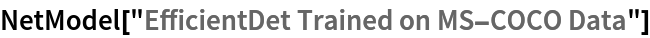Out=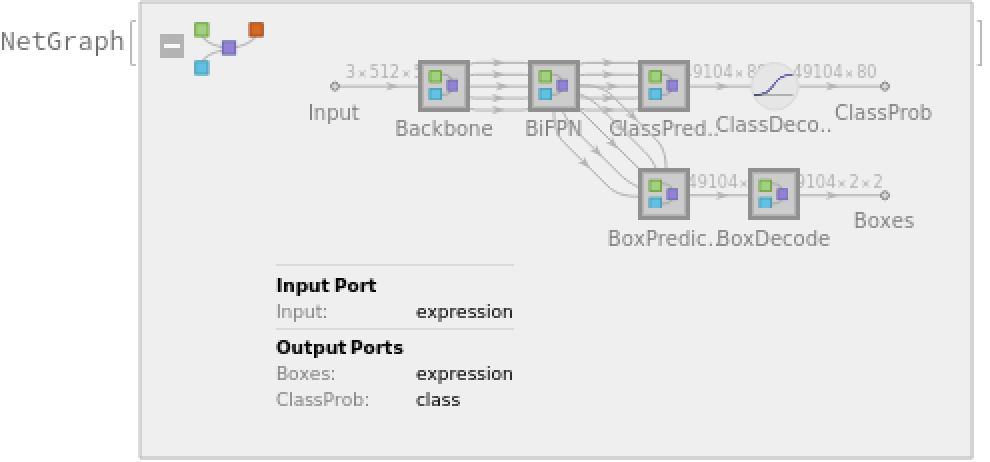### NetModel parameters

This model consists of a family of individual nets, each identified by a specific parameter combination. Inspect the available parameters:

 In:=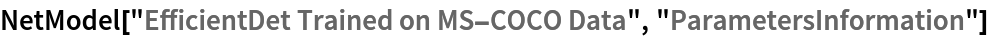Out=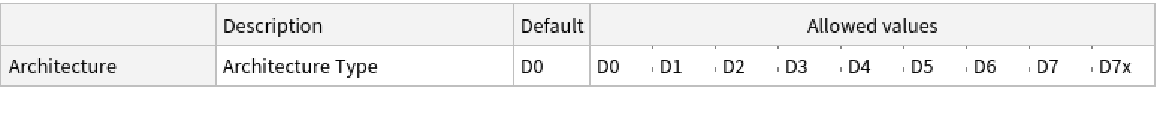Pick a non-default net by specifying the parameters:

 In:=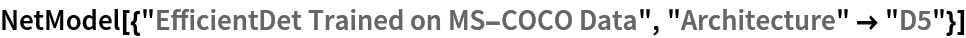Out=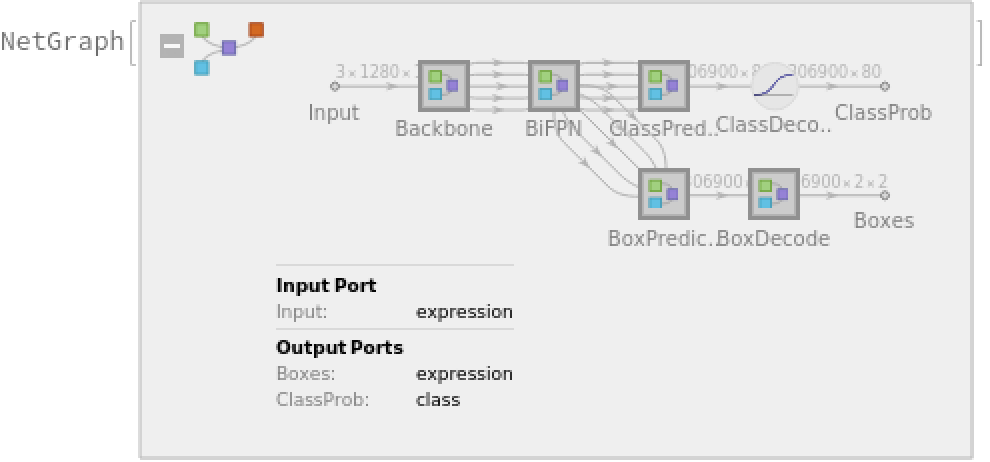Pick a non-default uninitialized net:

 In:=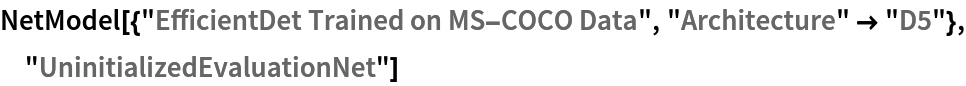Out=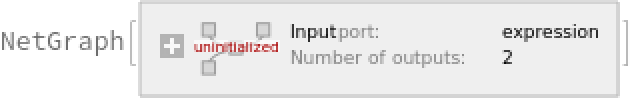### Evaluation function

Write an evaluation function to scale the result to the input image size and suppress the least probable detections:

 In:=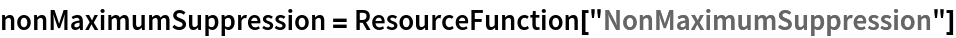Out=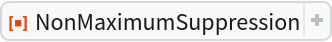In:=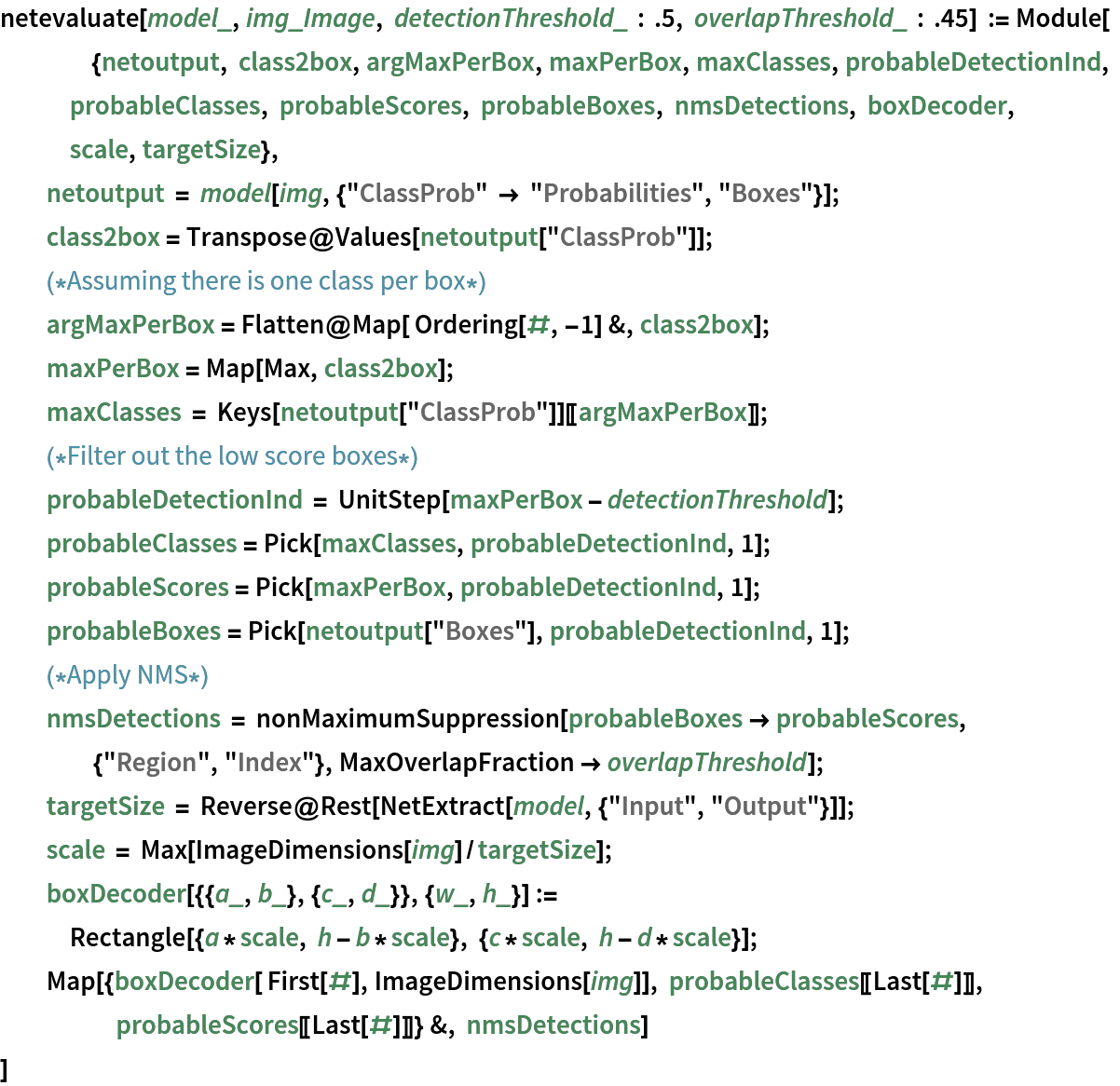### Basic usage

Obtain the detected bounding boxes with their corresponding classes and confidences for a given image:

 In:=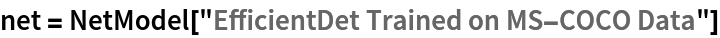Out=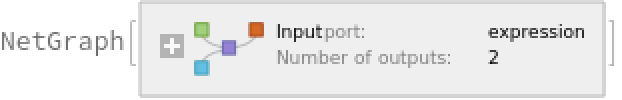In:=In:=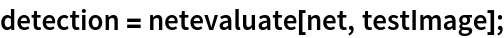Inspect which classes are detected:

 In:=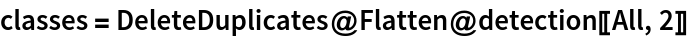Out=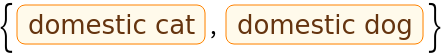Visualize the detection:

 In:=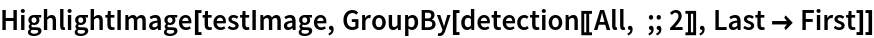Out=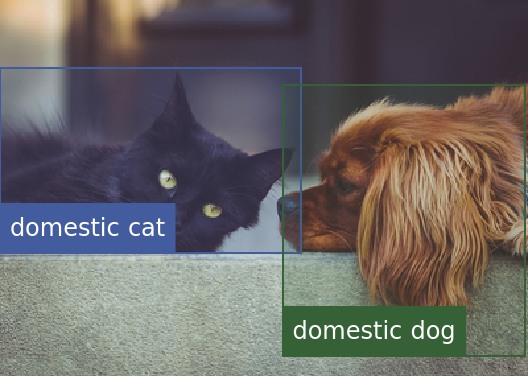### Network result

The network computes 49,104 bounding boxes and the probability that the objects in each box are of any given class:

 In:=In:=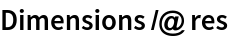Out=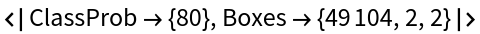In:=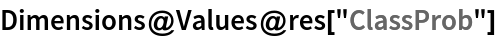Out=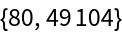Visualize all the boxes predicted by the net scaled by their "objectness" measures:

 In:=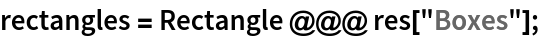In:=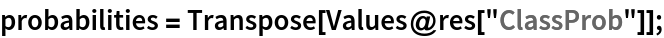In:=Out=Visualize all the boxes scaled by the probability that they contain a cat:

 In:=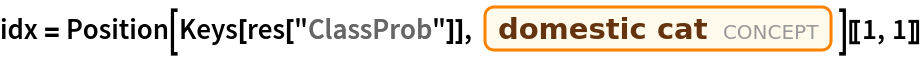Out=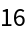In:=Out=Superimpose the cat prediction on top of the scaled input received by the net:

 In:=Out=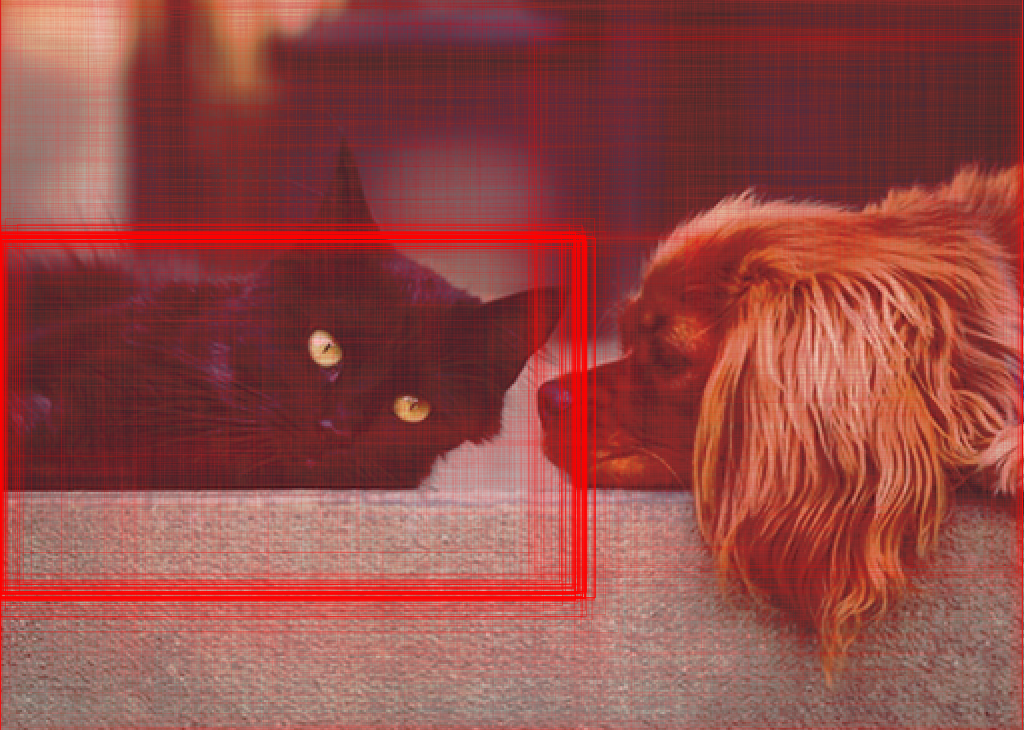### Net information

Inspect the number of parameters of all arrays in the net:

 In:=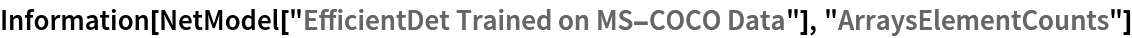Out=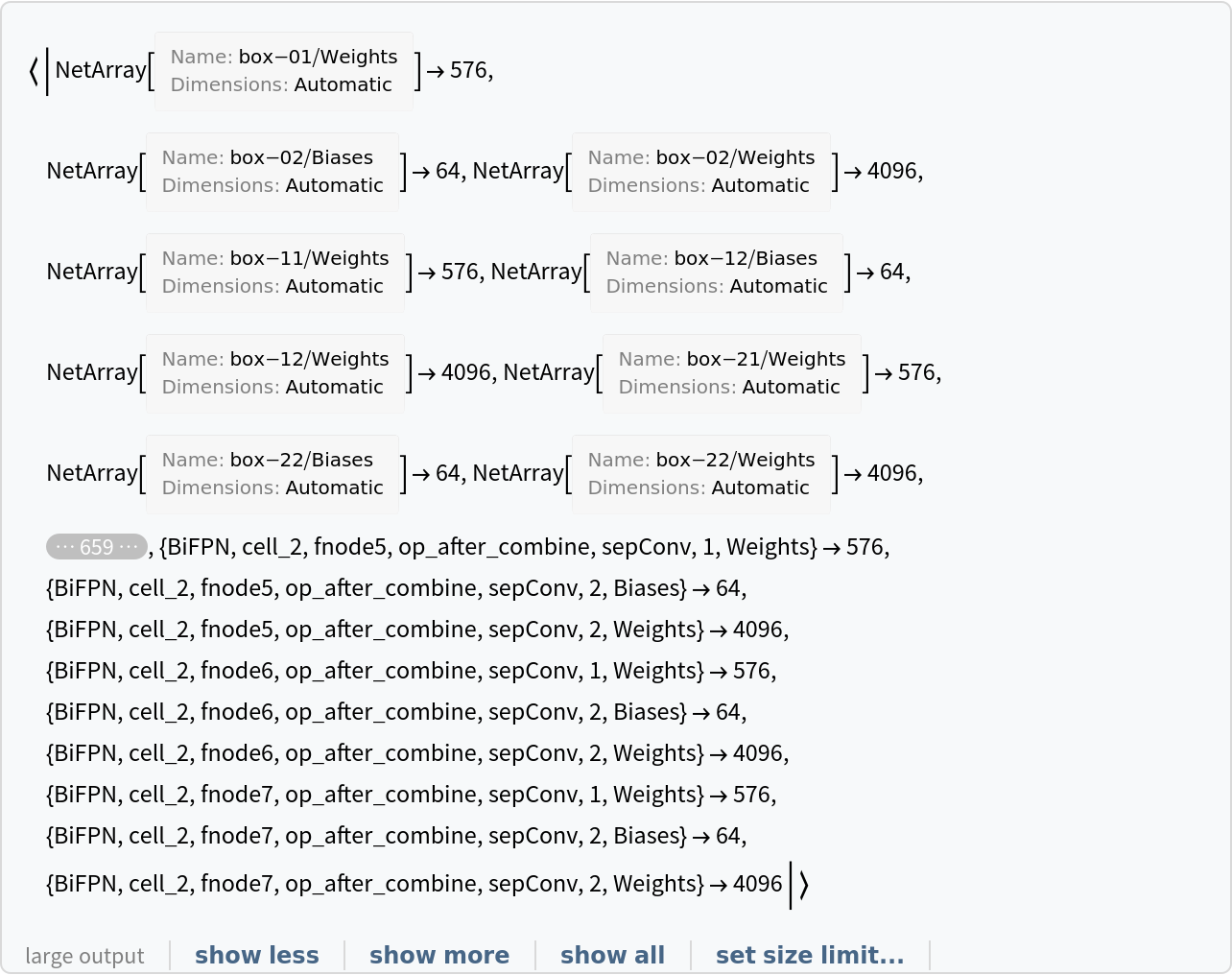Obtain the total number of parameters:

 In:=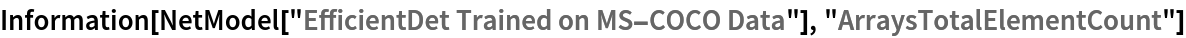Out=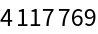Obtain the layer type counts:

 In:=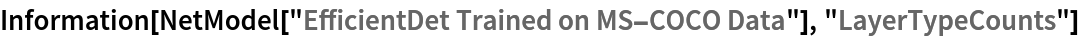Out=Display the summary graphic:

 In:=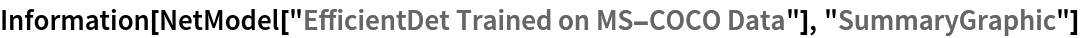In:=